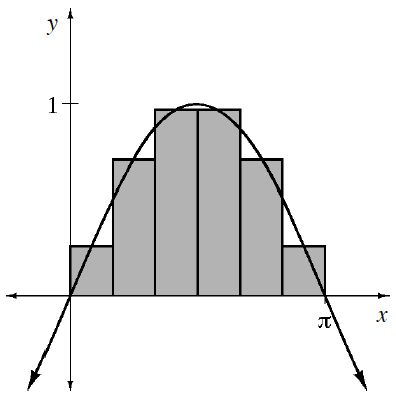### Home > APCALC > Chapter 2 > Lesson 2.2.1 > Problem2-55

2-55.

For $f (x) = \sin(x)$, an estimation of the area under the curve for $0\le x\le\pi$ is shown at right using six midpoint rectangles of equal width.

1. Estimate the area using these rectangles.

Base of each rectangle:

$\Delta x=\frac{\pi }{6}$

Height of each rectangle is determined by the function: $f(x)=\sin x$ evaluated at each midpoint.

Let's examine one of the rectangles, the smallest one:

$\text{Midpoint of smallest rectangle: }x= \frac{1}{2}\left ( \frac{\pi }{6} \right )=\frac{\pi }{12}$

$\text{Height of smallest rectangle: }y= \text{sin}\frac{\pi }{12}$

$\text{Area of smallest rectangle: }A= bh=\left ( \frac{\pi }{6} \right )\left ( \frac{\pi }{12} \right )=\left (\frac{\pi }{72} \right )$

Two medium sized rectangles with midpoints at ____ and ____.
Two large rectangles with midpoints at ____ and ____.
Find the area of all six rectangles.

Notice there are two small rectangles with midpoints at

$\frac{\pi }{12}\text{ and } \frac{11\pi }{12}.$

2. If the shaded region is rotated about the x-axis, then each of these rectangles becomes what shape? Sketch a picture representing this situation.

Imagine a barbell with six cylindrical weight: two small, two medium and two large. Each cylinder has a thickness (or height) of

$\frac{\pi }{6},$

but their circular bases vary in sizes. The radius of each circular base is determined by the function $f(x)=\sin x$ evaluated at

$x=\frac{\pi }{12}, \frac{3\pi }{12}, \frac{5\pi }{12}, \frac{7\pi }{12}, \frac{9\pi }{12}, \frac{11\pi }{12}.$

The smallest cylinders are on the outside, then medium then large.

3. Estimate the volume of this rotated region by calculating the volume of each of the rotated rectangles.

Because of symmetry, you can evaluate the volume of three of the cylinders and then double the result.

Volume of a cylinder: $V = \pi r^2h = \pi r^2(\Delta x)$